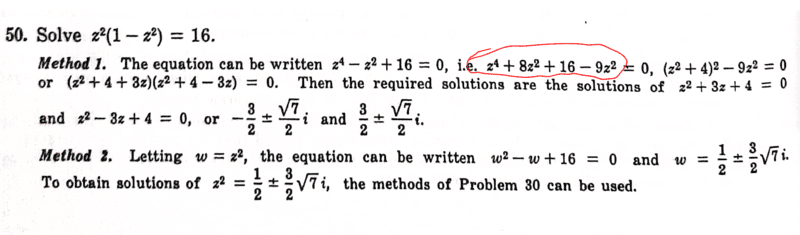# Solve ##z^2(1-z^2)=16## using Complex numbers

• chwala
In summary, the conversation discusses finding the formula for ##A^2=B^2## by manipulating the equation ##z^4+8z^2+16-9z^2=0## using the complete square method. It also mentions a systematic approach for factorizing the equation and solving for the coefficients. Another approach is introduced by substituting ##w=z^2## and comparing the real and imaginary parts to obtain the solution. The purpose of this manipulation is to fit the equation into the desired form of ##z^4+8z^2+16-9z^2##.

#### chwala

Gold Member
Homework Statement
See attached
Relevant Equations
Complex numbers
The problem is as shown...all steps are pretty easy to follow. I need help on the highlighted part in red. How did they come to;
##z^4+8z^2+16-9z^2=0## or is it by manipulating ##-z^2= 8z^2-9z^2?## trial and error ...The idea is to make formula of
$$A^2=B^2$$
. Let us see.
$$z^4+16=z^2$$
$$(z^2+4)^2=9z^2=(3z)^2$$
$$z^2+4=\pm 3z$$

A systematic approach is to factorize $$z^4 - z^2 + 16 = (z^2 + Az + 4)(z^2 + Bz + 4)$$ and compare coefficients of powers of $z$ to get three equations for $A$ and $B$: $$\begin{split} z^3 \quad &: \quad A + B = 0 \\ z^2 \quad &: \quad 8 + AB = -1 \\ z \quad &: \quad 4A + 4B = 0 \end{split}$$ Since the first and third are really the same equation, we can solve this system to obtain $(A, B) = (3, -3)$.

•PeroK and chwala
chwala said:
How did they come to

completion of the square

•fresh_42
malawi_glenn said:
completion of the square
How did they come to ##z^4+8z^2+16-9z^2##?...of course the step after is complete square method...no problem there. Or it was just simply identifying that;

##-9z^2+8z^2=-z^2##

Last edited:
•malawi_glenn
chwala said:
How did they come to ##z^4+8z^2+16-9z^2##?
##z^4-z^2+16 = z^4-z^2+16 + 0 = z^4-z^2+16 +8z^2 - 8z^2 = z^4+8z^2+16-9z^2 ##

•chwala
malawi_glenn said:
##z^4-z^2+16 = z^4-z^2+16 + 0 = z^4-z^2+16 +8z^2 - 8z^2 = z^4+8z^2+16-9z^2 ##
@malawi_glenn smart move there mate!

Here's another approach. Let ##w = z^2##:$$ww^* = |w|^2 = 16\frac{|w|^2}{16} = w(1-w)\frac{|w|^2}{16}$$ $$\Rightarrow w^* = (1-w)\frac{|w|^2}{16}$$Comparing the real and imaginary parts with ##w = a + ib## gives:$$-b = -b\frac{|w|^2}{16} \Rightarrow |w|^2 = 16 \Rightarrow a^2 + b^2 = 16$$$$a = 1-a \Rightarrow a = \frac 1 2 \Rightarrow b^2 = -\frac{63}{4}$$$$\Rightarrow z^2 = \frac 1 2 \pm \frac{3\sqrt 7}{2}i$$

••chwala and malawi_glenn
chwala said:
@malawi_glenn smart move there mate!
And you do this in order to complete the square adding and subtracting the same thing so it fits

•chwala